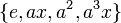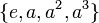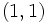# Subgroup structure of dihedral group:D8

View subgroup structure of particular groups | View other specific information about dihedral group:D8

The dihedral group$D_8$, sometimes called$D_4$, also called the dihedral group of order eight or the dihedral group acting on four elements, is defined by the following presentation:$\langle x,a| a^4 = x^2 = e, xax^{-1} = a^{-1}\rangle$

In the permutation representation, we can think of the dihedral group as a subgroup of the symmetric group on the four-element set$\{ 1,2,3,4\}$, and write:$a = (1,2,3,4), \qquad x = (1,3)$.

## Tables for quick information

### Table classifying subgroups up to automorphisms

FACTS TO CHECK AGAINST FOR SUBGROUP STRUCTURE: (group of prime power order)
Lagrange's theorem (order of subgroup times index of subgroup equals order of whole group, so all subgroups have prime power orders)|order of quotient group divides order of group (and equals index of corresponding normal subgroup, so all quotients have prime power orders)
prime power order implies not centerless | prime power order implies nilpotent | prime power order implies center is normality-large
size of conjugacy class of subgroups divides index of center
congruence condition on number of subgroups of given prime power order: The total number of subgroups of any fixed prime power order is congruent to 1 mod the prime.

In the "List of subgroups" columns below, a row break within the cell indicates that each row represents one conjugacy class of subgroups.

Automorphism class of subgroups List of subgroups Isomorphism class Order of subgroups Index of subgroups Number of conjugacy classes (=1 iff automorph-conjugate subgroup) Size of each conjugacy class (=1 iff normal subgroup) Total number of subgroups (=1 iff characteristic subgroup) Isomorphism class of quotient (if subgroup is normal) Subnormal depth (if proper and normal, this equals 1) Nilpotency class
trivial subgroup$\{ e \}$ trivial group 1 8 1 1 1 dihedral group:D8 1 0
center$\{ e,a^2 \}$ cyclic group:Z2 2 4 1 1 1 Klein four-group 1 1
other subgroups of order two$\{e,x \}, \{ e,a^2x \}$$\{ e,ax \}, \{ e,a^3x \}$
cyclic group:Z2 2 4 2 2 4 -- 2 1
Klein four-subgroups$\{ e,x,a^2,a^2x \}$,$\{ e,ax,a^2,a^3x \}$ Klein four-group 4 2 2 1 2 cyclic group:Z2 1 1
cyclic maximal subgroup$\{ e,a,a^2,a^3 \}$ cyclic group:Z4 4 2 1 1 1 cyclic group:Z2 1 1
whole group$\{ e,a,a^2,a^3,x,ax,a^2x,a^3x \}$ dihedral group:D8 8 1 1 1 1 trivial group 0 2
Total (6 rows) -- -- -- -- 8 -- 10 -- -- --

### Table classifying isomorphism types of subgroups

The first part of the GAP ID is the order of the group.

Group name GAP ID Index Occurrences as subgroup Conjugacy classes of occurrence as subgroup Automorphism classes of occurrence as subgroup Occurrences as normal subgroup Occurrences as characteristic subgroup
Trivial group$(1,1)$ 8 1 1 1 1 1
Cyclic group:Z2$(2,1)$ 4 5 3 2 1 1
Cyclic group:Z4$(4,1)$ 2 1 1 1 1 1
Klein four-group$(4,2)$ 2 2 2 1 2 0
Dihedral group:D8$(8,3)$ 1 1 1 1 1 1
Total -- -- 10 8 6 6 4

### Table listing number of subgroups by order

Note that these orders satisfy the congruence condition on number of subgroups of given prime power order: the number of subgroups of order$p^r$ is congruent to$1$ modulo$p$. Here,$p = 2$, so this means that the number of subgroups of any given order is odd.

Group order Index Occurrences as subgroup Conjugacy classes of occurrence as subgroup Automorphism classes of occurrences as subgroup Occurrences as normal subgroup Occurrences as characteristic subgroup
1 8 1 1 1 1 1
2 4 5 3 2 1 1
4 2 3 3 2 3 1
8 1 1 1 1 1 1
Total -- 10 8 6 6 4

## Defining functions

### Subgroup-defining functions and associated quotient-defining functions

Subgroup-defining function What it means Value as subgroup Value as group Order Associated quotient-defining function Value as group Order (= index of subgroup)
center elements that commute with every group element center of dihedral group:D8:$\{ e, a^2 \}$ cyclic group:Z2 2 inner automorphism group Klein four-group 4
derived subgroup subgroup generated by all commutators center of dihedral group:D8:$\{ e, a^2 \}$ cyclic group:Z2 2 abelianization Klein four-group 4
Frattini subgroup intersection of all maximal subgroups center of dihedral group:D8:$\{ e, a^2 \}$ cyclic group:Z2 2 Frattini quotient Klein four-group 4
Jacobson radical intersection of all maximal normal subgroups center of dihedral group:D8:$\{ e, a^2 \}$ cyclic group:Z2 2  ? Klein four-group 4
socle join of all minimal normal subgroups center of dihedral group:D8:$\{ e, a^2 \}$ cyclic group:Z2 2  ? Klein four-group 4
Fitting subgroup join of all nilpotent normal subgroups whole group dihedral group:D8 8 Fitting quotient trivial group 1
join of abelian subgroups of maximum order join of all abelian subgroups of maximum order among abelian subgroups whole group dihedral group:D8 8  ? trivial group 1
join of abelian subgroups of maximum rank join of all abelian subgroups of maximum rank among abelian subgroups whole group dihedral group:D8 8  ? trivial group 1
join of elementary abelian subgroups of maximum order join of all elementary abelian subgroups of maximum order among elementary abelian subgroups whole group dihedral group:D8 8  ? trivial group 1
ZJ-subgroup center of the join of abelian subgroups of maximum order center of dihedral group:D8:$\{ e, a^2 \}$ cyclic group:Z2 2  ? Klein four-group 4

### Subgroup series-defining functions

Series-defining function Type Zeroth member First member Second member Third member Stable member
upper central series ascending trivial center:$\{ e, a^2 \}$ -- center of dihedral group:D8 second center: whole group whole group whole group
lower central series descending -- whole group derived subgroup:$\{ e, a^2 \}$ -- center of dihedral group:D8 trivial trivial
derived series descending whole group derived subgroup:$\{ e, a^2 \}$ -- center of dihedral group:D8 trivial trivial trivial
Frattini series descending whole group Frattini subgroup:$\{ e, a^2 \}$ -- center of dihedral group:D8 trivial trivial trivial
Fitting series ascending trivial Fitting subgroup: whole group whole group whole group whole group
socle series ascending trivial socle:$\{ e, a^2 \}$ -- center of dihedral group:D8 whole group whole group whole group

## Lattice of subgroups

### The entire lattice

The lattice of subgroups of the dihedral group has the following interesting features:

• Since the group$D_8$ has no nontrivial power automorphisms, all the automorphisms of$D_8$ act nontrivially on the lattice. The inner automorphism group, which has order four, contains the identity automorphism, an automorphism that flips the two left-most order two subgroup, an automorphism that flips the two right-most order two subgroups, and an automorphism that does both flips. Note that inner automorphisms preserve all the order four subgroups. The outer automorphisms (which form exactly one coset of the inner automorphism group) exchange the two normal Klein four-subgroups that are not characteristic, while preserving the cyclic characteristic order four subgroup.
• Every automorphism of the lattice does arise from a group automorphism. Combined with the previous point, we get that the automorphism group of the subgroup lattice is isomorphic to the automorphism group of$D_8$.
• The lattice does not enjoy reverse symmetry, i.e., it is not equal to its opposite lattice. This is because there are five subgroups of order two, as compared to three subgroups of order four.

### The lattice collapsed to conjugacy classes

If we collapse the lattice under the equivalence relation of conjugacy, we find that the 2-subnormal subgroups contained in the same normal subgroup of order four collapse into single entities. The new diagram obtained is considerably simpler, and the inner automorphism group acts trivially on it. The outer automorphism group simply flips the two conjugacy classes of 2-subnormal subgroups and the two normal non-characteristic subgroups. These are the only automorphisms of this lattice, so the automorphism group of this lattice is isomorphic to the outer automorphism group of$D_8$.

### The sublattice of normal subgroups

For the sublattice of normal subgroups, we delete the four 2-subnormal subgroups of order two, leaving only the center. The center is the unique minimal normal subgroup (i.e., a monolith) and is contained in three maximal normal subgroups of order four. Note that this lattice is isomorphic to the lattice of normal subgroups of the quaternion group, but the quaternion group has no non-normal subgroups.

Some aspects of this generalize to arbitrary$p$-groups: if the center is of order$p$ it is the unique minimal normal subgroup. In fact, more general results include: prime power order implies center is normality-large, minimal normal implies central in nilpotent, omega-1 of center is normality-large in nilpotent p-group. The upshot of all these results is that the minimal normal subgroups of a finite$p$-group are precisely the subgroups of order$p$ in the center.

The lattice of normal subgroups is isomorphic to that for the quaternion group. In fact, these are both groups having the same Hall-Senior genus, namely$8\Gamma_2a$. The picture is given by:

### The sublattice of characteristic subgroups

The sublattice of characteristic subgroups is totally ordered, comprising the identity, the center, the four-element cyclic subgroup, and the whole group. Note that there is one characteristic subgroup of every order dividing the group order. This differs from the quaternion group case, which has no characteristic subgroup of order four.

### The sublattice of fully invariant subgroups

The sublattice of fully invariant subgroups comprises the identity element, the center, and the whole group. It is totally ordered.

### Abelian subgroups and elementary abelian subgroups

The abelian subgroups are obtained simply by removing the whole group from the lattice, while the elementary abelian subgroups are obtained by removing the whole group and the cyclic subgroup of order four.

## Subgroups of subgroups

We look at the three main types of examples of subgroups$H \le K \le G$, where$G$ is the dihedral group of order eight,$K$ is a subgroup of order four, and$H$ is a subgroup of order two.

### The center contained in the cyclic characteristic subgroup

In our notation,$H = \langle a^2 \rangle$ and$K = \langle a \rangle$.

Here, both$H$ and$K$ are characteristic in$G$. Also,$H$ is characteristic in$K$.

This gives examples for the following:

• Characteristicity does not satisfy image condition: Although$K$ is characteristic in$G$,$K/H$ is not characteristic in$G/H$.
• Intermediate characteristicity is not transitive
• Isomorph-freeness is not transitive

### The center contained in a Klein four-subgroup

There are two examples of this, equivalent via an outer automorphism. One is$H = \langle a^2 \rangle$ and$K = \langle a^2, x \rangle$. The other is$H = \langle a^2 \rangle$,$K = \langle a^2, ax \rangle$.

Here,$H$ is characteristic in$G$. However,$H$ is not characteristic in$K$ and$K$ is not characteristic in$G$.

This gives examples for the following:

### A 2-subnormal subgroup contained in a Klein four-subgroup

There are four examples of this, all related via automorphisms. One is$H = \langle x \rangle$,$K = \langle a^2, x \rangle$. This gives examples for the following:

## Intersecting pairs of subgroups

### A cyclic characteristic subgroup and Klein four-subgroup

Here,$H = \langle a \rangle$ and$K = \langle a^2, x \rangle$. This gives examples of the following:

### Two Klein four-subgroups

Here$H = \langle a^2, x \rangle$ and$K = \langle a^2, ax \rangle$. This gives examples of the following:

## Aspects of subgroup structure relevant for embeddings in bigger groups

### 2-automorphism-invariance and 2-core-automorphism-invariance

A subgroup of a$p$-group is termed a p-automorphism-invariant subgroup if it is invariant under all automorphisms of the whole group whose order is a power of$p$, while it is termed a p-core-automorphism-invariant subgroup if it is invariant under all automorphisms in the$p$-core of the automorphism group. We have:

Characteristic$\implies$$p$-automorphism-invariant$\implies$$p$-core-automorphism-invariant$\implies$ normal

In the case of the dihedral group, we have the following:

• The characteristic subgroups are the same as the$2$-automorphism-invariant subgroups which in turn are the same as the$2$-core-automorphism-invariant subgroups, namely: the whole group, the trivial subgroup, the cyclic subgroup of order four, and the center. Thus, the only subgroups of the dihedral group that are normal in every$2$-group containing it are the whole group, the trivial subgroup, the cyclic subgroup of order four, and the center. In other words, for each of the elementary abelian subgroups of order four, we can find bigger$2$-groups containing the dihedral group in which these are not normal.
• Also of note is the following: the dihedral group of order eight has an elementary abelian subgroup of order four but no elementary abelian$2$-core-automorphism-invariant subgroup of order four. This gives, indirectly, an example of a group having an elementary abelian subgroup of order four but no elementary abelian normal subgroup of order four. Namely, we need to find a bigger group that contains the$2$-core of the automorphism group of the dihedral group, acting on the dihedral group by inner automorphisms. The smallest such example is dihedral group:D16.

### 2-normal-extensible automorphism-invariance and normal-extensible automorphism-invariance

It turns out that for the dihedral group of order eight, every$2$-automorphism (and hence, every automorphism) is$2$-normal extensible, and hence the$2$-normal-extensible automorphism-invariant subgroups are precisely the same as the characteristic subgroups. In particular, the two non-characteristic normal subgroups of order four are not$2$-normal-extensible automorphism-invariant.

More generally, it turns out that since the center of the dihedral group has order two, and the inner automorphism group is maximal in the automorphism group, every automorphism is a normal-extensible automorphism. In particular, the normal-extensible automorphism-invariant subgroups are the same as the characteristic subgroups, and thus, the two non-characteristic normal subgroups of order four are not normal-extensible automorphism-invariant.

It follows that there is no group containing the dihedral group of order eight as a normal subgroup, such that one of these subgroups becomes a characteristic subgroup.

### Coprime automorphism-invariance

Since the automorphism group of this group is also a$2$-group, every subgroup is coprime automorphism-invariant. In particular, every normal subgroup is a coprime automorphism-invariant subgroup, and every normal subgroup of order four is an isomorph-normal coprime automorphism-invariant subgroup. We can deduce the following:

• For any group containing the dihedral group of order eight as a$2$-Sylow subgroup, all the subgroups of order$1,4,8$ are weakly closed subgroups.
• On the other hand, none of the subgroups of order two are weakly closed subgroups. Clearly, the non-normal subgroups are not weakly closed. Even the center, which is a normal subgroup, need not be weakly closed. The best example is the symmetric group of degree four, which has three conjugate$2$-Sylow subgroups, all dihedral of order eight, intersecting in a normal Klein four-group comprising the double transpositions. The centers of the three subgroups are the two-element subgroups of this Klein four-group. There are inner automorphisms of the whole group that interchange these groups, and hence their centers, which are all inside this normal Klein four-group. However, there is no inner automorphism in the normalizer of the dihedral Sylow subgroup that sends its center to any of the other centers.

## Maximality notions related to abelianness

### Abelian subgroups of maximum order

There are three abelian subgroups of maximum order: a cyclic characteristic subgroup of order four (type (5)), and the two elementary abelian subgroups of order four (type (4)). These are the only subgroups that are maximal among abelian subgroups.

The join of abelian subgroups of maximum order, sometimes called the Thompson subgroup and denoted by$J$, is thus the whole group. In particular, the ZJ-subgroup, which is the center of this Thompson subgroup, is simply the center of the whole group, i.e., the subgroup of type (2) in the listing.

### Abelian subgroups of maximum rank

The rank of the dihedral group is two, and there are two abelian subgroups of maximum rank. These are the two elementary abelian subgroups of order four (type (4)) and they are automorphic subgroups. The join of abelian subgroups of maximum rank is the whole group.

### Elementary abelian subgroups of maximum order

These coincide with the abelian subgroups of maximum rank.

### Centrally large subgroups

It turns out that all nontrivial normal subgroups of the group are centralizer-large subgroups, and the three subgroups of order four as well as the whole group are centrally large subgroups. The minimal CL-subgroups are thus the subgroups of order four, and their common commutator subgroup is the center (type (2)). The unique largest centrally large subgroup is the whole group.

### Subgroups with abelianization of maximum order

Further information: Subgroup with abelianization of maximum order

These are again the three subgroups of order four and the whole group. All of these have abelianizations of order four.